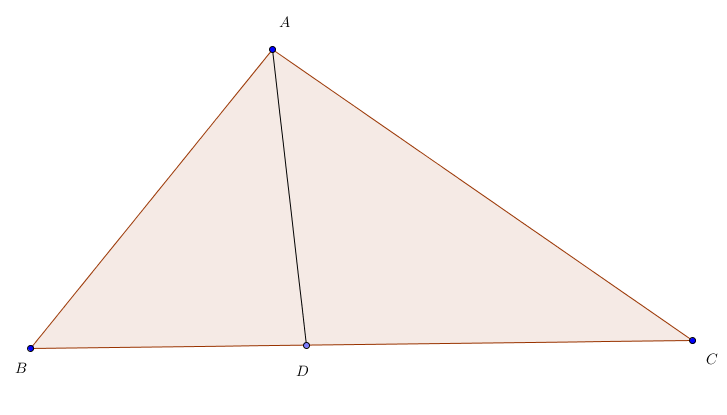# RMO 2001; Part - 1

Geometry Level 3In $\Delta ABC$, $D$ is a point on $BC$ such that $AD$ is the internal angle bisector of $\angle A$. Suppose $\angle B = 2 \angle C$ & $CD = AB$, then find the measure of $\angle A$ in degrees.

×